# sklearn.model_selection.GridSearchCV¶

class sklearn.model_selection.GridSearchCV(estimator, param_grid, scoring=None, n_jobs=None, iid=’warn’, refit=True, cv=’warn’, verbose=0, pre_dispatch=‘2*n_jobs’, error_score=’raise-deprecating’, return_train_score=False)[source]

Exhaustive search over specified parameter values for an estimator.

Important members are fit, predict.

GridSearchCV implements a “fit” and a “score” method. It also implements “predict”, “predict_proba”, “decision_function”, “transform” and “inverse_transform” if they are implemented in the estimator used.

The parameters of the estimator used to apply these methods are optimized by cross-validated grid-search over a parameter grid.

Read more in the User Guide.

Parameters:
estimator : estimator object.

This is assumed to implement the scikit-learn estimator interface. Either estimator needs to provide a score function, or scoring must be passed.

param_grid : dict or list of dictionaries

Dictionary with parameters names (string) as keys and lists of parameter settings to try as values, or a list of such dictionaries, in which case the grids spanned by each dictionary in the list are explored. This enables searching over any sequence of parameter settings.

scoring : string, callable, list/tuple, dict or None, default: None

A single string (see The scoring parameter: defining model evaluation rules) or a callable (see Defining your scoring strategy from metric functions) to evaluate the predictions on the test set.

For evaluating multiple metrics, either give a list of (unique) strings or a dict with names as keys and callables as values.

NOTE that when using custom scorers, each scorer should return a single value. Metric functions returning a list/array of values can be wrapped into multiple scorers that return one value each.

See Specifying multiple metrics for evaluation for an example.

If None, the estimator’s score method is used.

n_jobs : int or None, optional (default=None)

Number of jobs to run in parallel. None means 1 unless in a joblib.parallel_backend context. -1 means using all processors. See Glossary for more details.

pre_dispatch : int, or string, optional

Controls the number of jobs that get dispatched during parallel execution. Reducing this number can be useful to avoid an explosion of memory consumption when more jobs get dispatched than CPUs can process. This parameter can be:

• None, in which case all the jobs are immediately created and spawned. Use this for lightweight and fast-running jobs, to avoid delays due to on-demand spawning of the jobs
• An int, giving the exact number of total jobs that are spawned
• A string, giving an expression as a function of n_jobs, as in ‘2*n_jobs’
iid : boolean, default=’warn’

If True, return the average score across folds, weighted by the number of samples in each test set. In this case, the data is assumed to be identically distributed across the folds, and the loss minimized is the total loss per sample, and not the mean loss across the folds. If False, return the average score across folds. Default is True, but will change to False in version 0.22, to correspond to the standard definition of cross-validation.

Changed in version 0.20: Parameter iid will change from True to False by default in version 0.22, and will be removed in 0.24.

cv : int, cross-validation generator or an iterable, optional

Determines the cross-validation splitting strategy. Possible inputs for cv are:

• None, to use the default 3-fold cross validation,
• integer, to specify the number of folds in a (Stratified)KFold,
• CV splitter,
• An iterable yielding (train, test) splits as arrays of indices.

For integer/None inputs, if the estimator is a classifier and y is either binary or multiclass, StratifiedKFold is used. In all other cases, KFold is used.

Refer User Guide for the various cross-validation strategies that can be used here.

Changed in version 0.20: cv default value if None will change from 3-fold to 5-fold in v0.22.

refit : boolean, string, or callable, default=True

Refit an estimator using the best found parameters on the whole dataset.

For multiple metric evaluation, this needs to be a string denoting the scorer that would be used to find the best parameters for refitting the estimator at the end.

Where there are considerations other than maximum score in choosing a best estimator, refit can be set to a function which returns the selected best_index_ given cv_results_.

The refitted estimator is made available at the best_estimator_ attribute and permits using predict directly on this GridSearchCV instance.

Also for multiple metric evaluation, the attributes best_index_, best_score_ and best_params_ will only be available if refit is set and all of them will be determined w.r.t this specific scorer. best_score_ is not returned if refit is callable.

See scoring parameter to know more about multiple metric evaluation.

Changed in version 0.20: Support for callable added.

verbose : integer

Controls the verbosity: the higher, the more messages.

error_score : ‘raise’ or numeric

Value to assign to the score if an error occurs in estimator fitting. If set to ‘raise’, the error is raised. If a numeric value is given, FitFailedWarning is raised. This parameter does not affect the refit step, which will always raise the error. Default is ‘raise’ but from version 0.22 it will change to np.nan.

return_train_score : boolean, default=False

If False, the cv_results_ attribute will not include training scores. Computing training scores is used to get insights on how different parameter settings impact the overfitting/underfitting trade-off. However computing the scores on the training set can be computationally expensive and is not strictly required to select the parameters that yield the best generalization performance.

Attributes:
cv_results_ : dict of numpy (masked) ndarrays

A dict with keys as column headers and values as columns, that can be imported into a pandas DataFrame.

For instance the below given table

param_kernel param_gamma param_degree split0_test_score rank_t…
‘poly’ 2 0.80 2
‘poly’ 3 0.70 4
‘rbf’ 0.1 0.80 3
‘rbf’ 0.2 0.93 1

will be represented by a cv_results_ dict of:

{
'param_kernel': masked_array(data = ['poly', 'poly', 'rbf', 'rbf'],
mask = [False False False False]...)
'param_gamma': masked_array(data = [-- -- 0.1 0.2],
mask = [ True  True False False]...),
'param_degree': masked_array(data = [2.0 3.0 -- --],
mask = [False False  True  True]...),
'split0_test_score'  : [0.80, 0.70, 0.80, 0.93],
'split1_test_score'  : [0.82, 0.50, 0.70, 0.78],
'mean_test_score'    : [0.81, 0.60, 0.75, 0.85],
'std_test_score'     : [0.01, 0.10, 0.05, 0.08],
'rank_test_score'    : [2, 4, 3, 1],
'split0_train_score' : [0.80, 0.92, 0.70, 0.93],
'split1_train_score' : [0.82, 0.55, 0.70, 0.87],
'mean_train_score'   : [0.81, 0.74, 0.70, 0.90],
'std_train_score'    : [0.01, 0.19, 0.00, 0.03],
'mean_fit_time'      : [0.73, 0.63, 0.43, 0.49],
'std_fit_time'       : [0.01, 0.02, 0.01, 0.01],
'mean_score_time'    : [0.01, 0.06, 0.04, 0.04],
'std_score_time'     : [0.00, 0.00, 0.00, 0.01],
'params'             : [{'kernel': 'poly', 'degree': 2}, ...],
}


NOTE

The key 'params' is used to store a list of parameter settings dicts for all the parameter candidates.

The mean_fit_time, std_fit_time, mean_score_time and std_score_time are all in seconds.

For multi-metric evaluation, the scores for all the scorers are available in the cv_results_ dict at the keys ending with that scorer’s name ('_<scorer_name>') instead of '_score' shown above. (‘split0_test_precision’, ‘mean_train_precision’ etc.)

best_estimator_ : estimator or dict

Estimator that was chosen by the search, i.e. estimator which gave highest score (or smallest loss if specified) on the left out data. Not available if refit=False.

See refit parameter for more information on allowed values.

best_score_ : float

Mean cross-validated score of the best_estimator

For multi-metric evaluation, this is present only if refit is specified.

best_params_ : dict

Parameter setting that gave the best results on the hold out data.

For multi-metric evaluation, this is present only if refit is specified.

best_index_ : int

The index (of the cv_results_ arrays) which corresponds to the best candidate parameter setting.

The dict at search.cv_results_['params'][search.best_index_] gives the parameter setting for the best model, that gives the highest mean score (search.best_score_).

For multi-metric evaluation, this is present only if refit is specified.

scorer_ : function or a dict

Scorer function used on the held out data to choose the best parameters for the model.

For multi-metric evaluation, this attribute holds the validated scoring dict which maps the scorer key to the scorer callable.

n_splits_ : int

The number of cross-validation splits (folds/iterations).

refit_time_ : float

Seconds used for refitting the best model on the whole dataset.

This is present only if refit is not False.

ParameterGrid
generates all the combinations of a hyperparameter grid.
sklearn.model_selection.train_test_split
utility function to split the data into a development set usable for fitting a GridSearchCV instance and an evaluation set for its final evaluation.
sklearn.metrics.make_scorer
Make a scorer from a performance metric or loss function.

Notes

The parameters selected are those that maximize the score of the left out data, unless an explicit score is passed in which case it is used instead.

If n_jobs was set to a value higher than one, the data is copied for each point in the grid (and not n_jobs times). This is done for efficiency reasons if individual jobs take very little time, but may raise errors if the dataset is large and not enough memory is available. A workaround in this case is to set pre_dispatch. Then, the memory is copied only pre_dispatch many times. A reasonable value for pre_dispatch is 2 * n_jobs.

Examples

>>> from sklearn import svm, datasets
>>> from sklearn.model_selection import GridSearchCV
>>> parameters = {'kernel':('linear', 'rbf'), 'C':[1, 10]}
>>> svc = svm.SVC(gamma="scale")
>>> clf = GridSearchCV(svc, parameters, cv=5)
>>> clf.fit(iris.data, iris.target)
...
GridSearchCV(cv=5, error_score=...,
estimator=SVC(C=1.0, cache_size=..., class_weight=..., coef0=...,
decision_function_shape='ovr', degree=..., gamma=...,
kernel='rbf', max_iter=-1, probability=False,
random_state=None, shrinking=True, tol=...,
verbose=False),
iid=..., n_jobs=None,
param_grid=..., pre_dispatch=..., refit=..., return_train_score=...,
scoring=..., verbose=...)
>>> sorted(clf.cv_results_.keys())
...
['mean_fit_time', 'mean_score_time', 'mean_test_score',...
'param_C', 'param_kernel', 'params',...
'rank_test_score', 'split0_test_score',...
'split2_test_score', ...
'std_fit_time', 'std_score_time', 'std_test_score']


Methods

 decision_function(self, X) Call decision_function on the estimator with the best found parameters. fit(self, X[, y, groups]) Run fit with all sets of parameters. get_params(self[, deep]) Get parameters for this estimator. inverse_transform(self, Xt) Call inverse_transform on the estimator with the best found params. predict(self, X) Call predict on the estimator with the best found parameters. predict_log_proba(self, X) Call predict_log_proba on the estimator with the best found parameters. predict_proba(self, X) Call predict_proba on the estimator with the best found parameters. score(self, X[, y]) Returns the score on the given data, if the estimator has been refit. set_params(self, \*\*params) Set the parameters of this estimator. transform(self, X) Call transform on the estimator with the best found parameters.
__init__(self, estimator, param_grid, scoring=None, n_jobs=None, iid=’warn’, refit=True, cv=’warn’, verbose=0, pre_dispatch=‘2*n_jobs’, error_score=’raise-deprecating’, return_train_score=False)[source]
decision_function(self, X)[source]

Call decision_function on the estimator with the best found parameters.

Only available if refit=True and the underlying estimator supports decision_function.

Parameters: X : indexable, length n_samples Must fulfill the input assumptions of the underlying estimator.
fit(self, X, y=None, groups=None, **fit_params)[source]

Run fit with all sets of parameters.

Parameters: X : array-like, shape = [n_samples, n_features] Training vector, where n_samples is the number of samples and n_features is the number of features. y : array-like, shape = [n_samples] or [n_samples, n_output], optional Target relative to X for classification or regression; None for unsupervised learning. groups : array-like, with shape (n_samples,), optional Group labels for the samples used while splitting the dataset into train/test set. Only used in conjunction with a “Group” cv instance (e.g., GroupKFold). **fit_params : dict of string -> object Parameters passed to the fit method of the estimator
get_params(self, deep=True)[source]

Get parameters for this estimator.

Parameters: deep : boolean, optional If True, will return the parameters for this estimator and contained subobjects that are estimators. params : mapping of string to any Parameter names mapped to their values.
inverse_transform(self, Xt)[source]

Call inverse_transform on the estimator with the best found params.

Only available if the underlying estimator implements inverse_transform and refit=True.

Parameters: Xt : indexable, length n_samples Must fulfill the input assumptions of the underlying estimator.
predict(self, X)[source]

Call predict on the estimator with the best found parameters.

Only available if refit=True and the underlying estimator supports predict.

Parameters: X : indexable, length n_samples Must fulfill the input assumptions of the underlying estimator.
predict_log_proba(self, X)[source]

Call predict_log_proba on the estimator with the best found parameters.

Only available if refit=True and the underlying estimator supports predict_log_proba.

Parameters: X : indexable, length n_samples Must fulfill the input assumptions of the underlying estimator.
predict_proba(self, X)[source]

Call predict_proba on the estimator with the best found parameters.

Only available if refit=True and the underlying estimator supports predict_proba.

Parameters: X : indexable, length n_samples Must fulfill the input assumptions of the underlying estimator.
score(self, X, y=None)[source]

Returns the score on the given data, if the estimator has been refit.

This uses the score defined by scoring where provided, and the best_estimator_.score method otherwise.

Parameters: X : array-like, shape = [n_samples, n_features] Input data, where n_samples is the number of samples and n_features is the number of features. y : array-like, shape = [n_samples] or [n_samples, n_output], optional Target relative to X for classification or regression; None for unsupervised learning. score : float
set_params(self, **params)[source]

Set the parameters of this estimator.

The method works on simple estimators as well as on nested objects (such as pipelines). The latter have parameters of the form <component>__<parameter> so that it’s possible to update each component of a nested object.

Returns: self
transform(self, X)[source]

Call transform on the estimator with the best found parameters.

Only available if the underlying estimator supports transform and refit=True.

Parameters: X : indexable, length n_samples Must fulfill the input assumptions of the underlying estimator.

## Examples using sklearn.model_selection.GridSearchCV¶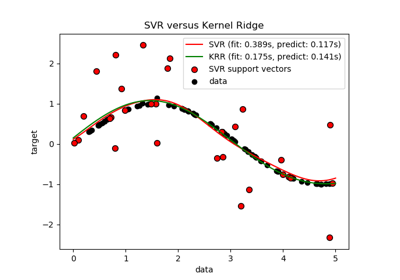Comparison of kernel ridge regression and SVRFaces recognition example using eigenfaces and SVMs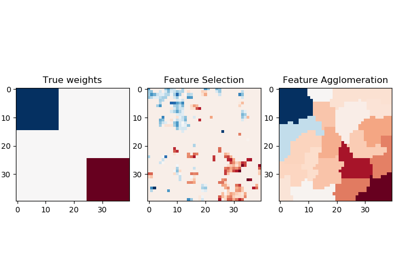Feature agglomeration vs. univariate selectionConcatenating multiple feature extraction methods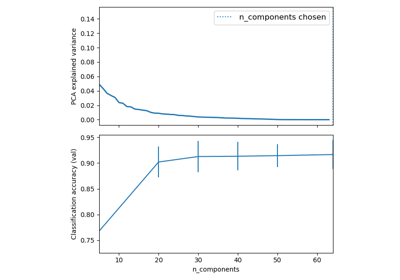Pipelining: chaining a PCA and a logistic regressionColumn Transformer with Mixed Types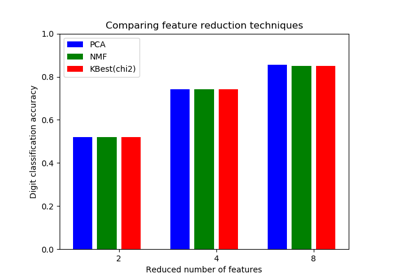Selecting dimensionality reduction with Pipeline and GridSearchCV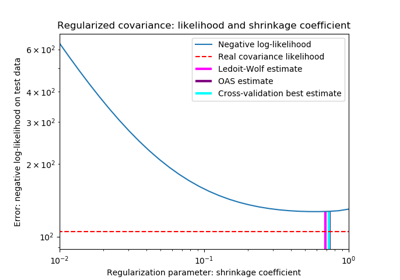Shrinkage covariance estimation: LedoitWolf vs OAS and max-likelihood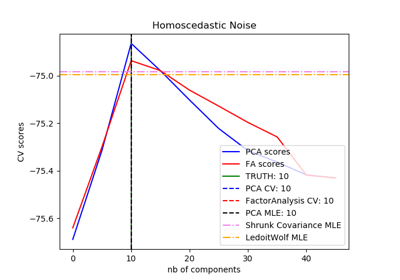Model selection with Probabilistic PCA and Factor Analysis (FA)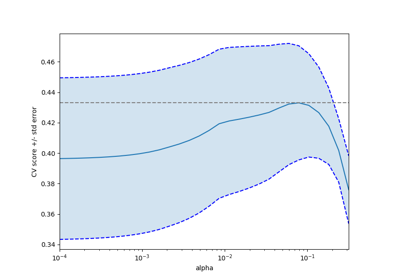Cross-validation on diabetes Dataset Exercise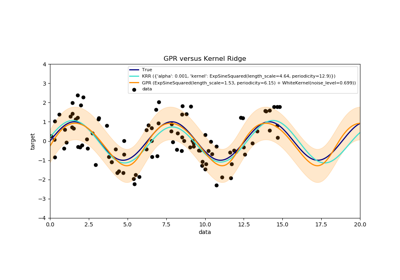Comparison of kernel ridge and Gaussian process regressionParameter estimation using grid search with cross-validationComparing randomized search and grid search for hyperparameter estimation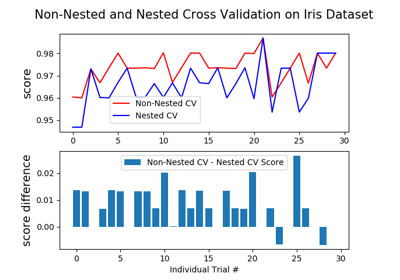Nested versus non-nested cross-validation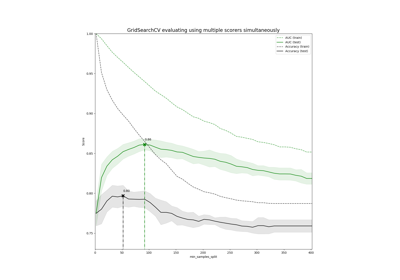Demonstration of multi-metric evaluation on cross_val_score and GridSearchCV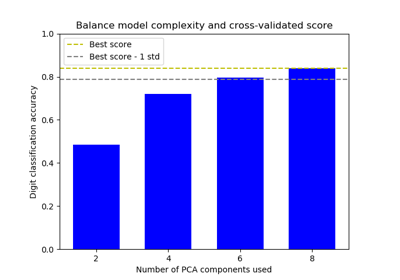Balance model complexity and cross-validated scoreSample pipeline for text feature extraction and evaluation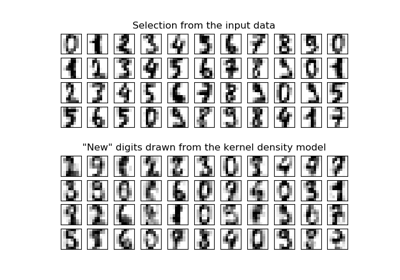Kernel Density Estimation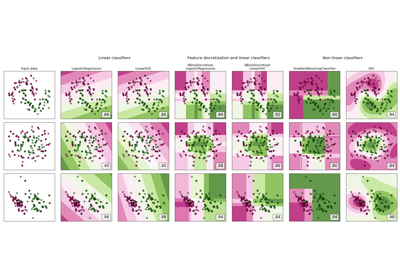Feature discretization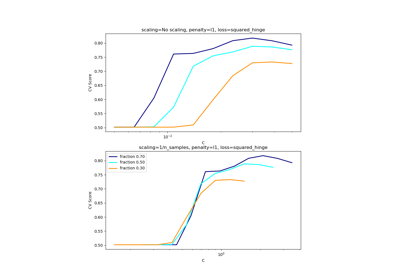Scaling the regularization parameter for SVCs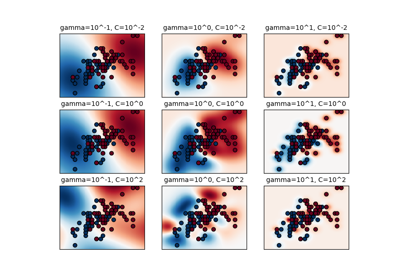RBF SVM parameters